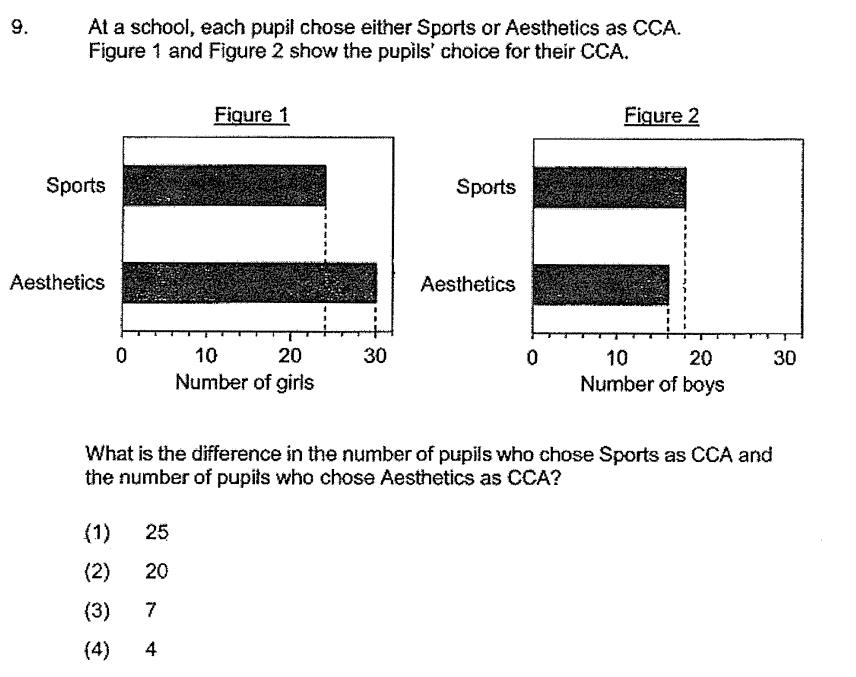### Still have math questions?

Arithmetic
Question9. At a school, each pupil chose either Sports or Aesthetics as CCA. Figure $$1$$ and Figure $$2$$ show the pupils' choice for their CCA. What is the difference in the number of pupils who chose Sports as CCA and the number of pupits who chose Aesthetics as CCA?

(1) $$25$$

(2) $$20$$

(3) $$7$$

(4) $$4$$

$$4$$